The function builds a neighbours list based on regions with contiguous boundaries, that is sharing one or more boundary point. The current function is in part interpreted and may run slowly for many regions or detailed boundaries, but from 0.2-16 should not fail because of lack of memory when single polygons are built of very many border coordinates.

poly2nb(pl, row.names = NULL, snap=sqrt(.Machine$double.eps), queen=TRUE, useC=TRUE, foundInBox=NULL) ## Arguments pl list of polygons of class extending SpatialPolygons, or an sf or sfc object containing non-empty (multi-)polygon objects row.names character vector of region ids to be added to the neighbours list as attribute region.id, default seq(1, nrow(x)); if pl has row.names, they are used instead of the default sequence. snap boundary points less than snap distance apart are considered to indicate contiguity; used both to find candidate and actual neighbours for planar geometries, but only actual neighbours for spherical geometries, as spherical spatial indexing itself injects some fuzzyness. queen if TRUE, a single shared boundary point meets the contiguity condition, if FALSE, more than one shared point is required; note that more than one shared boundary point does not necessarily mean a shared boundary line useC default TRUE, doing the work loop in C, may be set to false to revert to R code calling two C functions in an n*k work loop, where k is the average number of candidate neighbours foundInBox default NULL using R code or st_intersects() to generate candidate neighbours (using snap= if the geometries are not spherical); if not NULL (for legacy purposes) a list of length (n-1) with integer vectors of candidate neighbours (j > i) (as created by the poly_findInBoxGEOS function in rgeos for clean polygons) ## Value A neighbours list with class nb. See card for details of “nb” objects. ## Note From 0.5-8, the function includes faster bounding box indexing and other improvements contributed by Micah Altman. If a cluster is provided using set.ClusterOption, it will be used for finding candidate bounding box overlaps for exact testing for contiguity. Until 1.1-7, sf polygons included both start and end points, so could erroneously report queen neighbourhood where only rook was present, see https://github.com/r-spatial/spdep/issues/50. From 1.1-9 with sf 1.0-0, s2 is used in bounding box indexing internally when pl is in geographical coordinates. Because the topology engine of s2 differs from the use of GEOS for planar coordinates by sf, some output differences may be expected. Since treating spherical geometries as planar is also questionable, it is not clear whether spherical contiguous polygon neighbours should simply follow neighbours found by treating the geometries as planar https://github.com/r-spatial/s2/issues/125#issuecomment-864403372. However, current advice is not necessarily to use s2 for finding contiguity neighbours, or at least to check output. ## Author Roger Bivand Roger.Bivand@nhh.no with contributions from Micah Altman ## See also summary.nb, card ## Examples columbus <- st_read(system.file("shapes/columbus.shp", package="spData"), quiet=TRUE) col.gal.nb <- read.gal(system.file("weights/columbus.gal", package="spData")) coords <- st_coordinates(st_centroid(st_geometry(columbus))) xx <- poly2nb(as(columbus, "Spatial")) dxx <- diffnb(xx, col.gal.nb) plot(st_geometry(columbus), border="grey") plot(col.gal.nb, coords, add=TRUE) plot(dxx, coords, add=TRUE, col="red") title(main=paste("Differences (red) in Columbus GAL weights (black)", "and polygon generated queen weights", sep="\n"), cex.main=0.6)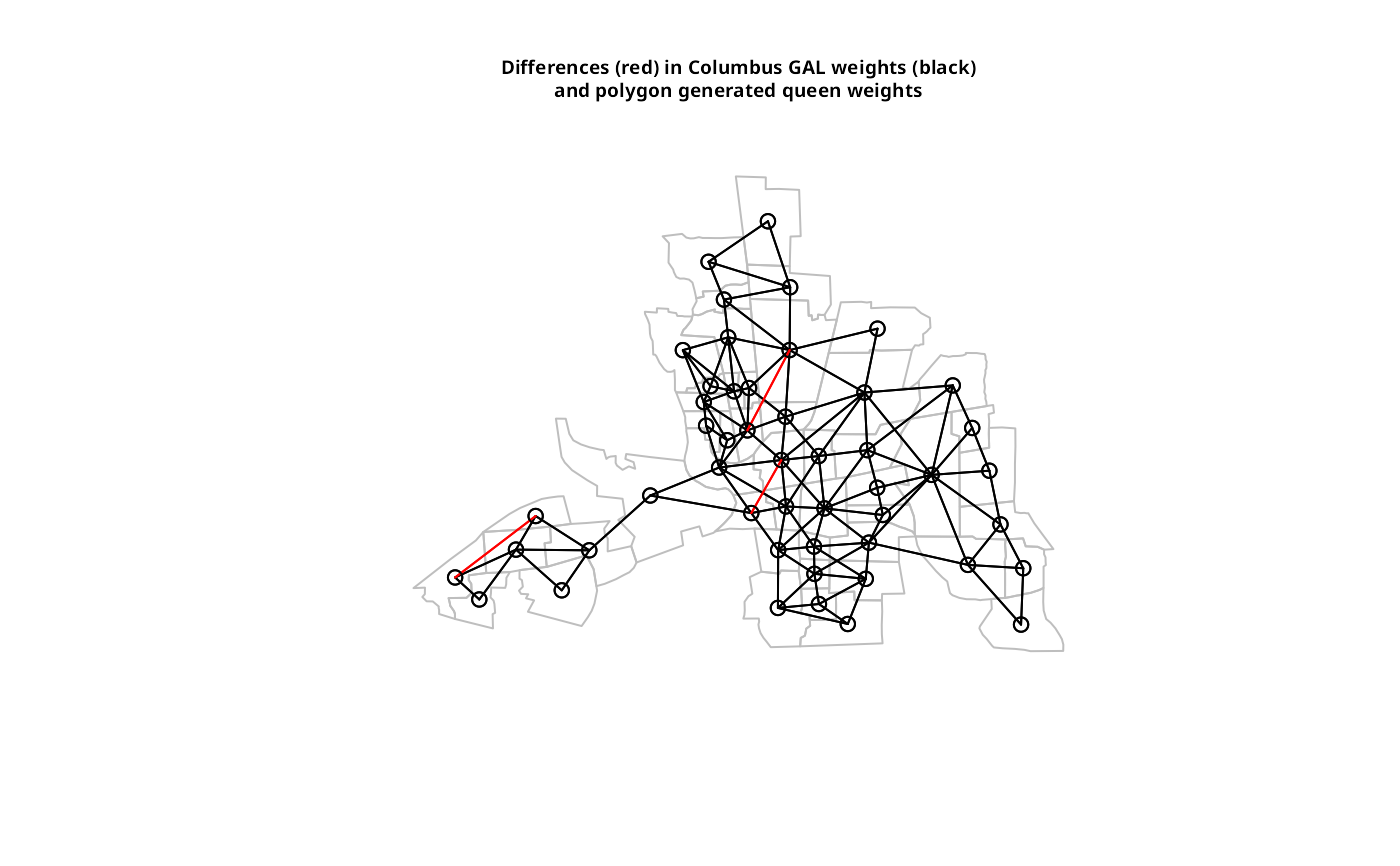# poly2nb with sf sfc_MULTIPOLYGON objects sf_xx <- poly2nb(columbus) diffnb(sf_xx, xx) #> Neighbour list object: #> Number of regions: 49 #> Number of nonzero links: 0 #> Percentage nonzero weights: 0 #> Average number of links: 0 #> 49 regions with no links: #> 1 2 3 4 5 6 7 8 9 10 11 12 13 14 15 16 17 18 19 20 21 22 23 24 25 26 27 #> 28 29 30 31 32 33 34 35 36 37 38 39 40 41 42 43 44 45 46 47 48 49 sfc_xx <- poly2nb(st_geometry(columbus)) diffnb(sfc_xx, xx) #> Neighbour list object: #> Number of regions: 49 #> Number of nonzero links: 0 #> Percentage nonzero weights: 0 #> Average number of links: 0 #> 49 regions with no links: #> 1 2 3 4 5 6 7 8 9 10 11 12 13 14 15 16 17 18 19 20 21 22 23 24 25 26 27 #> 28 29 30 31 32 33 34 35 36 37 38 39 40 41 42 43 44 45 46 47 48 49 xxx <- poly2nb(as(columbus, "Spatial"), queen=FALSE) dxxx <- diffnb(xxx, col.gal.nb) plot(st_geometry(columbus), border = "grey") plot(col.gal.nb, coords, add = TRUE) plot(dxxx, coords, add = TRUE, col = "red") title(main=paste("Differences (red) in Columbus GAL weights (black)", "and polygon generated rook weights", sep="\n"), cex.main=0.6)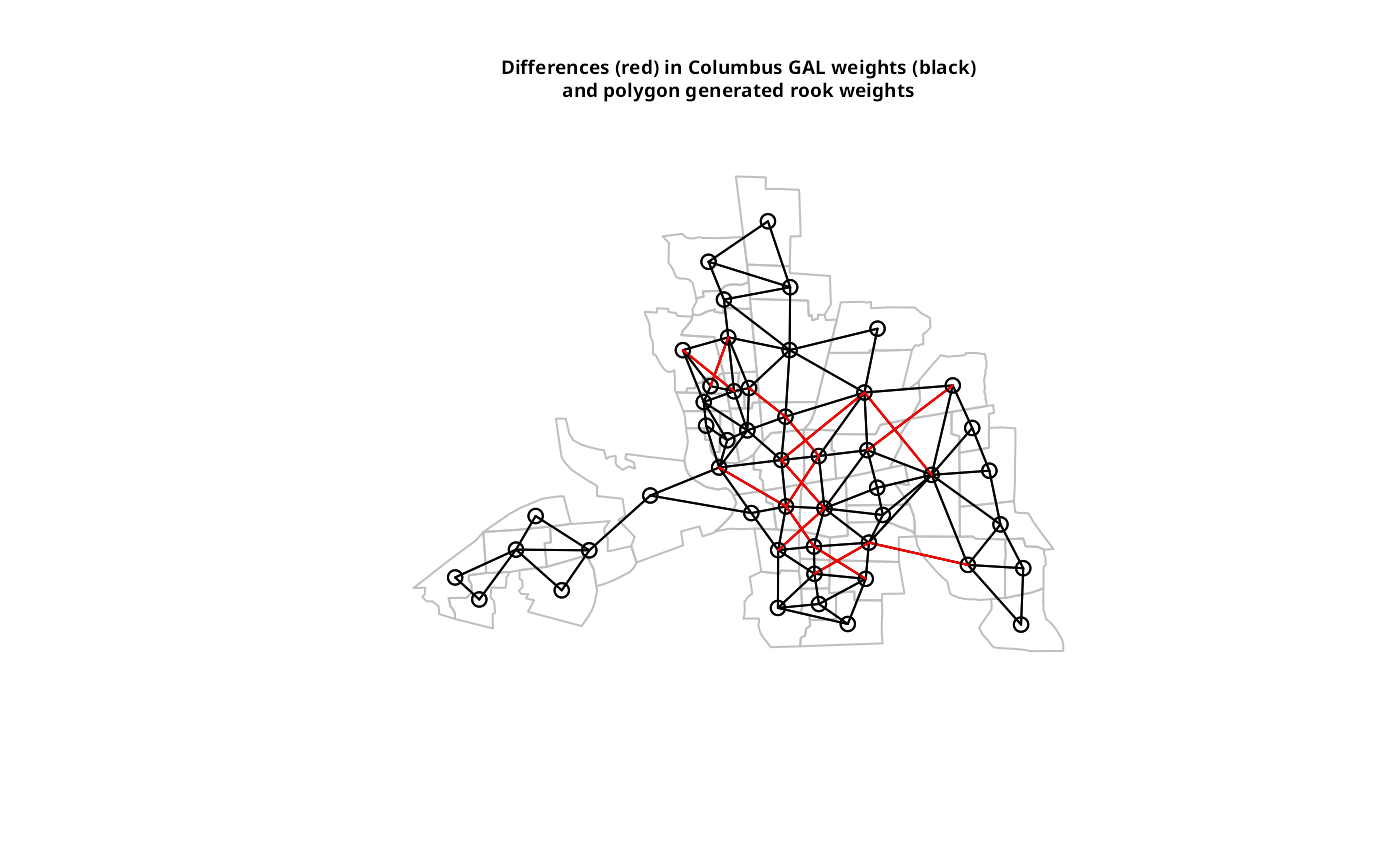cards <- card(xx) maxconts <- which(cards == max(cards)) if(length(maxconts) > 1) maxconts <- maxconts fg <- rep("grey", length(cards)) fg[maxconts] <- "red" fg[xx[[maxconts]]] <- "green" plot(st_geometry(columbus), col=fg) title(main="Region with largest number of contiguities", cex.main=0.6)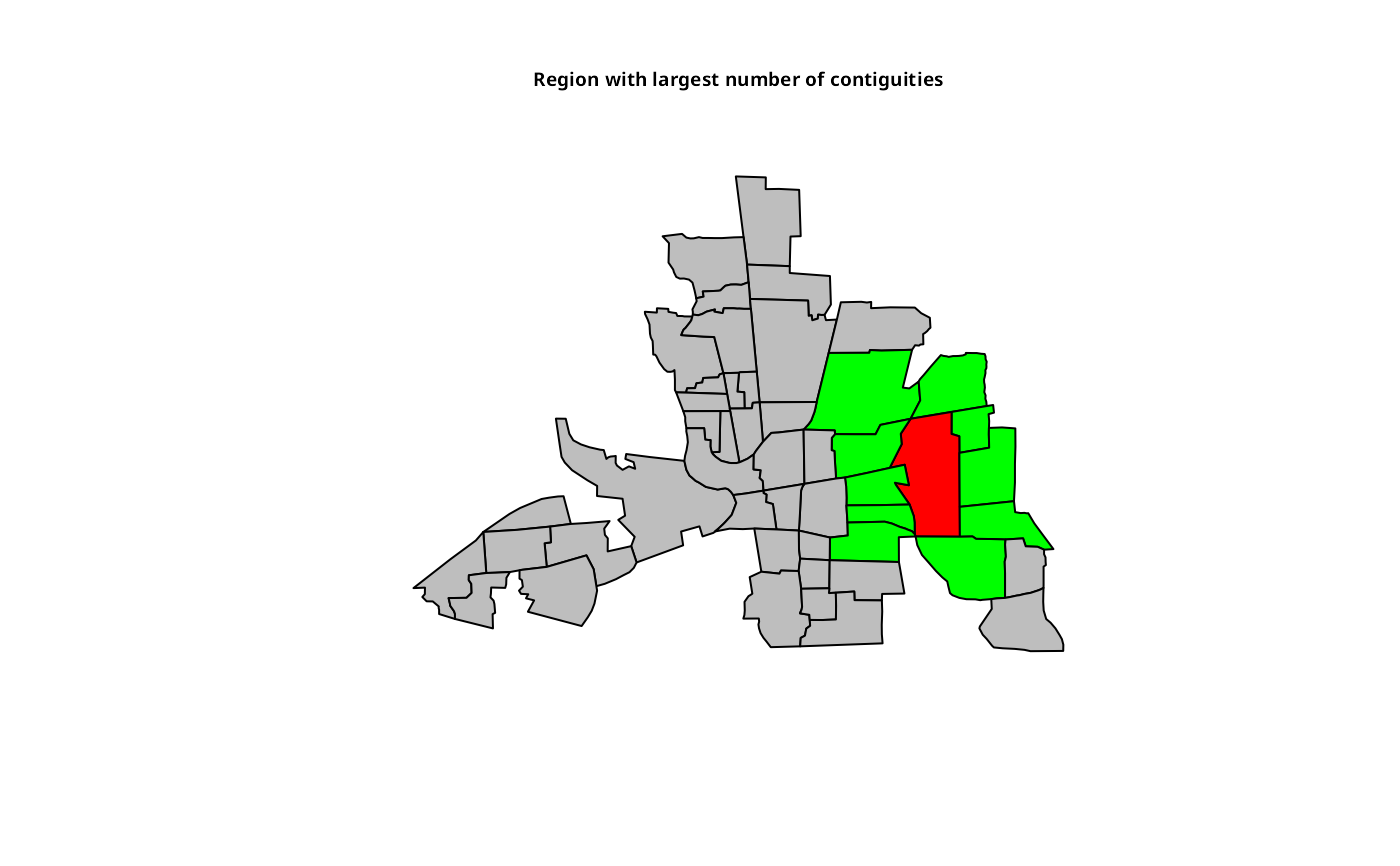nc.sids <- st_read(system.file("shapes/sids.shp", package="spData"), quiet=TRUE) system.time(xxnb <- poly2nb(nc.sids)) #> user system elapsed #> 0.020 0.001 0.021 system.time(xxnb <- poly2nb(as(nc.sids, "Spatial"))) #> user system elapsed #> 0.04 0.00 0.04 plot(st_geometry(nc.sids)) plot(xxnb, coordinates(as(nc.sids, "Spatial")), add=TRUE, col="blue")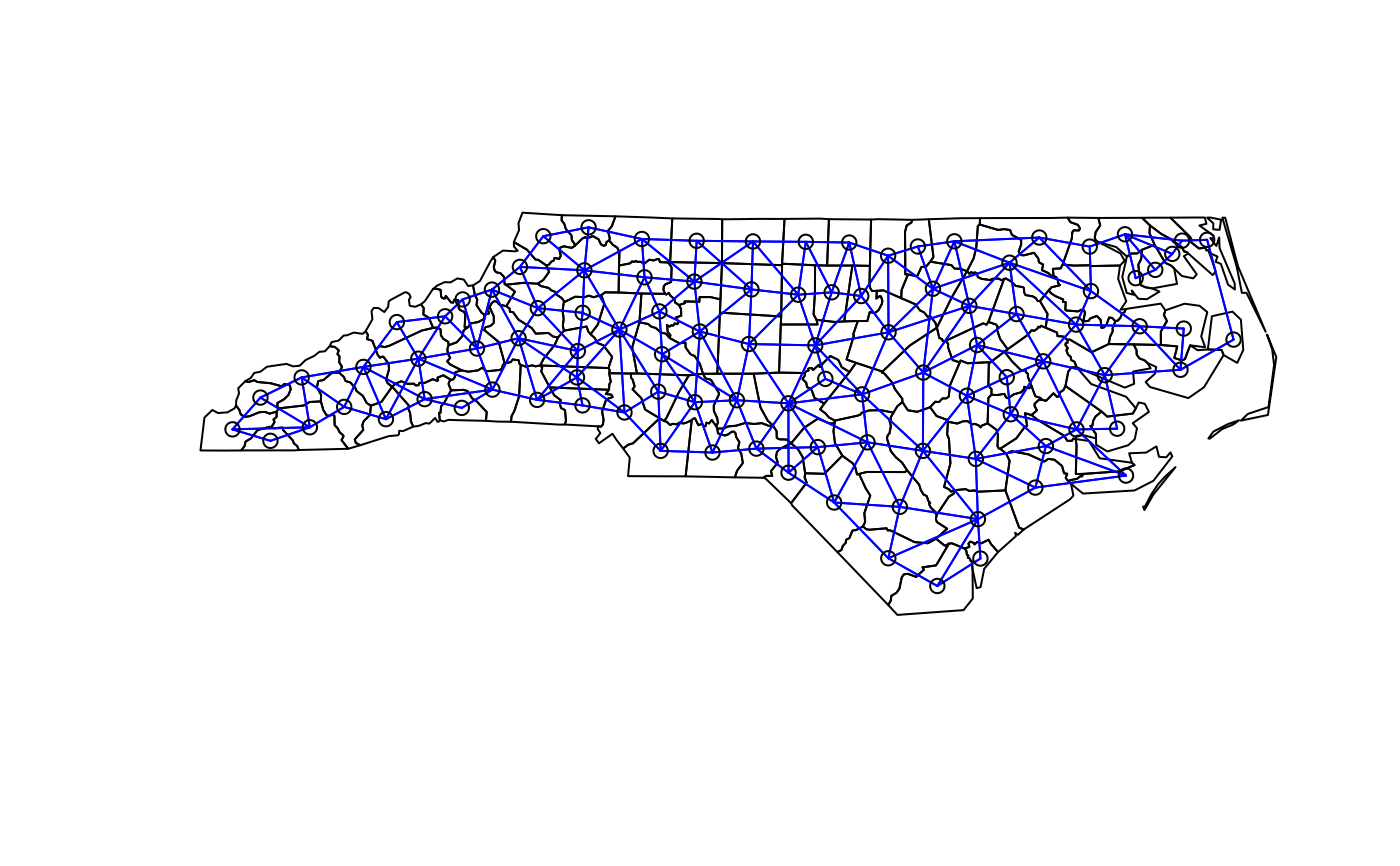sq <- st_polygon(list(rbind(c(0,0), c(1,0), c(1,1), c(0,1), c(0,0)))) sq2 <- sq + c(0,1) sq3 <- sq + c(1,0) sq4 <- sq + c(1,1) gm <- st_sfc(list(sq, sq2, sq3, sq4)) df <- st_as_sf(gm, id=1:4) plot(st_geometry(df)) text(st_coordinates(st_centroid(gm)), as.character(df$id))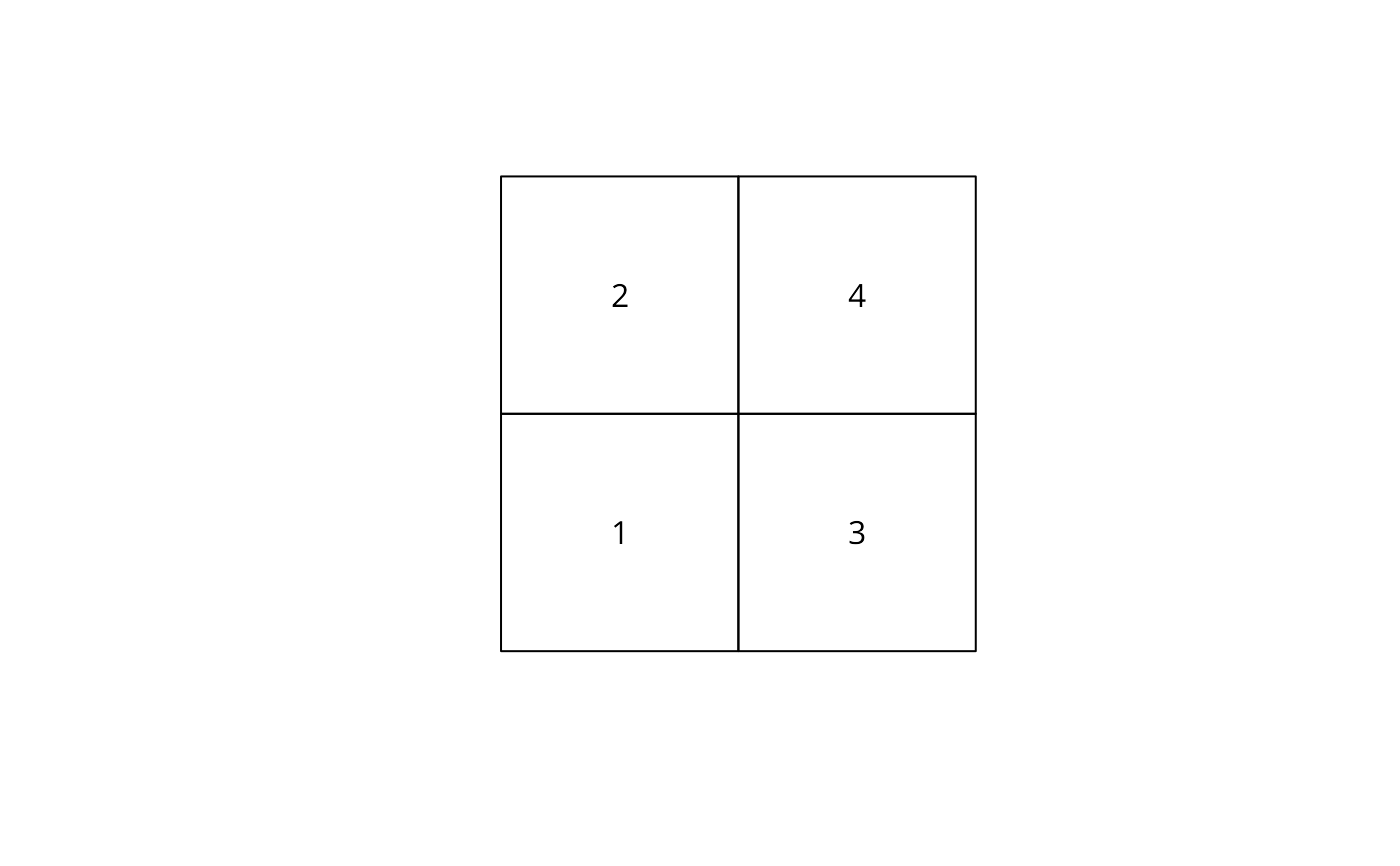unclass(poly2nb(df, queen = FALSE))
#> []
#>  2 3
#>
#> []
#>  1 4
#>
#> []
#>  1 4
#>
#> []
#>  2 3
#>
#> attr(,"region.id")
#>  "1" "2" "3" "4"
#> attr(,"call")
#> poly2nb(pl = df, queen = FALSE)
#> attr(,"type")
#>  "rook"
#> attr(,"sym")
#>  TRUE